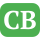CppBuzz.com

# What is the difference between C++ Map and Multimap

• The multimap stores pairs of (key,value) where both key and value can appear several times
• The map > will only store each value once for a specific key. To do that, it will have to be able to compare the values, not just the keys
• A std::map is an associative container, that allows you to have a unique key associated with your type value

## Example of Map in C++

void someFunction() { // declare Map typedef std::map<std::string, int> MapType; mapType myMap; // insertion myMap.insert(MapType::value_type("test", 42)); myMap.insert(MapType::value_type("other-test", 0)); // search auto it = myMap.find("test"); if (it != myMap.end()) std::cout<<"value for"<<it->first<<"is"<<it->second<<std::endl; else std::cout << "value not found" << std::endl; }

• A std::multimap is equal to a std::map, but your keys are not unique anymore. Therefore you can find a range of items instead of just find one unique item.

## Example of Multimap in C++

void someFunction() { // declare Multimap typedef std::multimap<std::string, int> MapType; mapType myMap; // insertion myMap.insert(MapType::value_type("test", 42)); myMap.insert(MapType::value_type("test", 45)); myMap.insert(MapType::value_type("other-test", 0)); // search std::pair<auto first, auto second> range = myMap.equal_range("test"); for (auto it = range.first; it != range.second; ++it) std::cout<<"value for "<<it->first<<"can be"<<it->second<<std::endl; }

• The std::set is like an std::map, but it is not storing a key associated to a value. It stores only the key type, and assures you that it is unique within the set.
• You also have the std::multiset, that follows the same pattern.
• All these containers provide an O(log(n)) access with their find / equal_range
• Because map containers do not allow for duplicate key values, the insertion operation checks for each element inserted whether another element exists already in the container with the same key value, if so, the element is not inserted and its mapped value is not changed in any way.
• With 1 TB of input, I would use neither. Most likely, you don't have enough RAM. Use some on disk data structure like B-tree.

[Project using Map & Multimap with Threads]